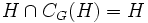Proving that a subgroup is self-centralizing

This is a survey article related to:self-centralizing subgroup
View other survey articles about self-centralizing subgroup

This article is about general strategies to prove that a subgroup (either a particular chosen subgroup, or a subgroup satisfying certain constraints) is self-centralizing.

For a list of examples/statements about subgroups being self-centralizing, check out:

The definition of self-centralizing subgroup

A subgroup$H$ of a group$G$ is termed self-centralizing in$G$ if it satisfies the following equivalent conditions, where$C_G(H)$ denotes the centralizer of$H$ in$G$ and$Z(H)$ denotes the center of$H$:

1.$C_G(H) \le H$
2.$C_G(H) = H \cap C_G(H)$
3.$C_G(H) = Z(H)$

In general, for any subgroup$H$ of$G$, the intersection$H \cap C_G(H)$ is the center$Z(H)$. In the special case where$H$ is Abelian,$H \cap C_G(H) = H$, or equivalently$H \le C_G(H)$. In this case, proving that$H$ is self-centralizing is equivalent to proving that$H = C_G(H)$.

The typical way we prove a subgroup is self-centralizing is using a maximality condition. Namely, we show that if$C_G(H)$ strictly contains$Z(H)$ (or, when$H$ is Abelian, strictly contains$H$), then we can find some subgroup between$Z(H)$ and$C_G(H)$ that violates a maximality assumption.

Maximality assumptions

Maximal among Abelian normal implies self-centralizing

The following results are true:

In other words, in a nilpotent or supersolvable group, if$H$ is an Abelian normal subgroup of$G$, and there is no Abelian normal subgroup of$G$ properly containing$H$, then$C_G(H) = H$, so$H$ is self-centralizing.

The key idea here is as follows. Since$H$ is Abelian,$H \le C_G(H)$.$C_G(H)$ is a bigger normal subgroup -- the problem is that it need not be Abelian. We use the nilpotence or supersolvability of$G$, to argue that we can find a cyclic normal subgroup of$G/H$ inside$C_G(H)/H$, and take the inverse image of this to get an Abelian normal subgroup properly containing$H$. This contradicts the maximality of$H$.

Thus, the key idea here is to find an intermediate subgroup -- not necessarily the whole of$C_G(H)$, that contradicts the maximality assumptions about$H$.

Fitting subgroup is self-centralizing in a solvable group

Further information: Fitting subgroup is self-centralizing in solvable

If$F(G)$ is the Fitting subgroup of$G$, we want to show that$C_G(F(G)) \le F(G)$. We do this by a similar method to the earlier problem: we first assume that$C_G(F(G))$ strictly contains the intersection$C_G(F(G)) \cap F(G)$. Note that the intersection is contained in the center of$C_G(F(G))$. We next use solvability to find a subgroup just slightly bigger than$C_G(F(G)) \cap F(G)$, such that its derived subgroup is contained in$C_G(F(G)) \cap F(G)$. This turns out to be a characteristic subgroup of nilpotence class two, and hence must be contained in$F(G)$ -- a contradiction.

Finding useful results

To prove a result about a subgroup being self-centralizing, we typically work by contradiction, as described above. To show that$C_G(H) = Z(H)$, we assume otherwise, and then try to find some subgroup inside$C_G(H)$, properly containing$Z(H)$, that yields a contradiction. Typical steps here are:

1. Instead of looking at$G$, we try to work in the quotient$G/Z(H)$. This step makes sense if we are given that$H$ is a normal subgroup. Indeed, for all the examples that we have here, the subgroup that we're trying to prove is self-centralizing, is already known to be normal.
2. Next, we try to work out what property a subgroup$K$ between$Z(H)$ and$C_G(H)$ should have, that'll contradict the maximality of$H$, and that can be expressed in terms of the quotient group$K/H$ as a subgroup of$G/H$. In the maximal among Abelian normal subgroups case, this was the property of being a cyclic normal subgroup inside the image of the centralizer. In the Fitting subgroup case, this was the property of being a subgroup leaving a nilpotent quotient.
3. Finally, we see what global information or conditions on the group can guarantee the existence of such a subgroup. In the maximal among Abelian normal subgroups case, nilpotence and supersolvability both guaranteed that every nontrivial normal subgroup contains a cyclic normal subgroup. In the Fitting subgroup case, solvability guaranteed the existence of a penultimate term to the derived series.

Sometimes, the search for such results may lead to modified, more complicated constructions. Some examples are described below.

Combining maximality with other information

Critical subgroup theorem

Further information: Thompson's critical subgroup theorem

One part of Thompson's critical subgroup theorem states that the characteristic subgroup constructed in a particular manner, is indeed a self-centralizing subgroup. For the proof of this, we first use the maximality assumptions to determine what the center of this characteristic subgroup should precisely be. Next, we assume that the centralizer strictly contains this center. Then, we put bounds on the centralizer, and finally show that its intersection with a certain subgroup is trivial -- something that cannot happen because that subgroup is normality-large: its intersection with every nontrivial normal subgroup is nontrivial.

The crucial idea here is to:

1. First get a precise hold on the center of the subgroup
2. Assume that the centralizer is bigger, and contradict a certain subgroup being normality-large

Central product decomposition lemma

Further information: central product decomposition lemma for characteristic rank one